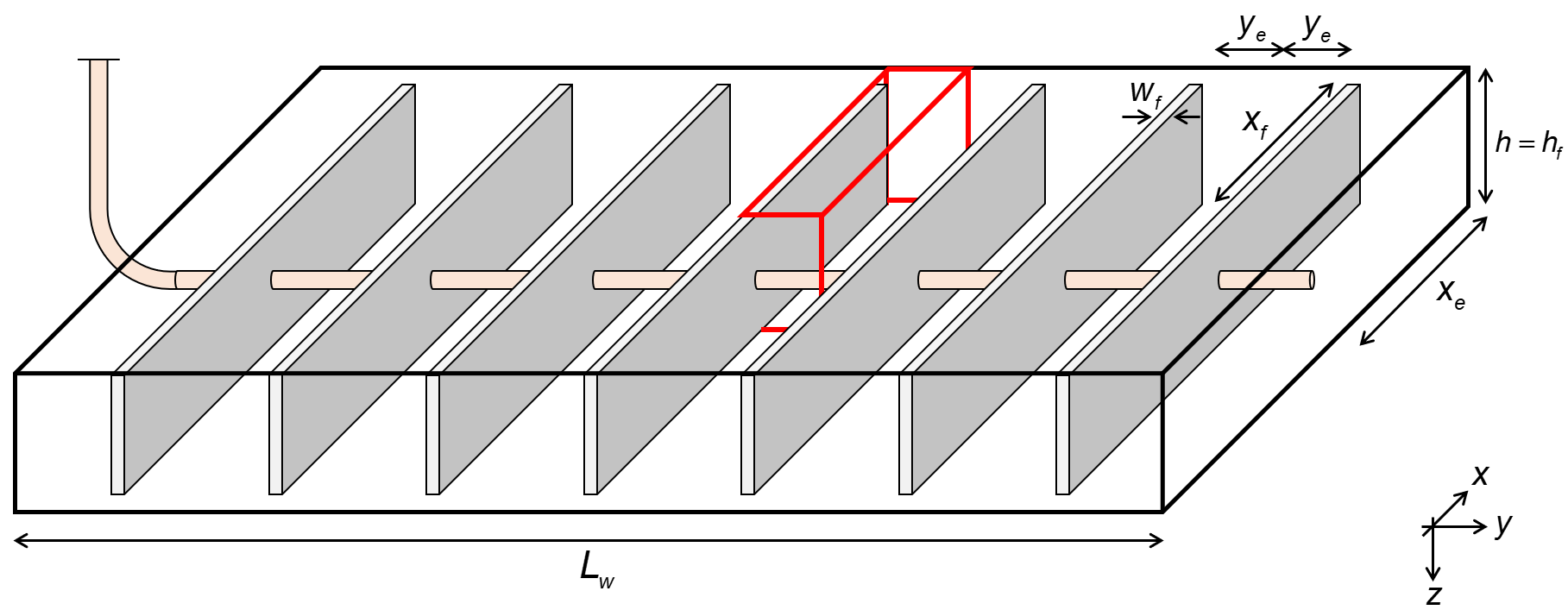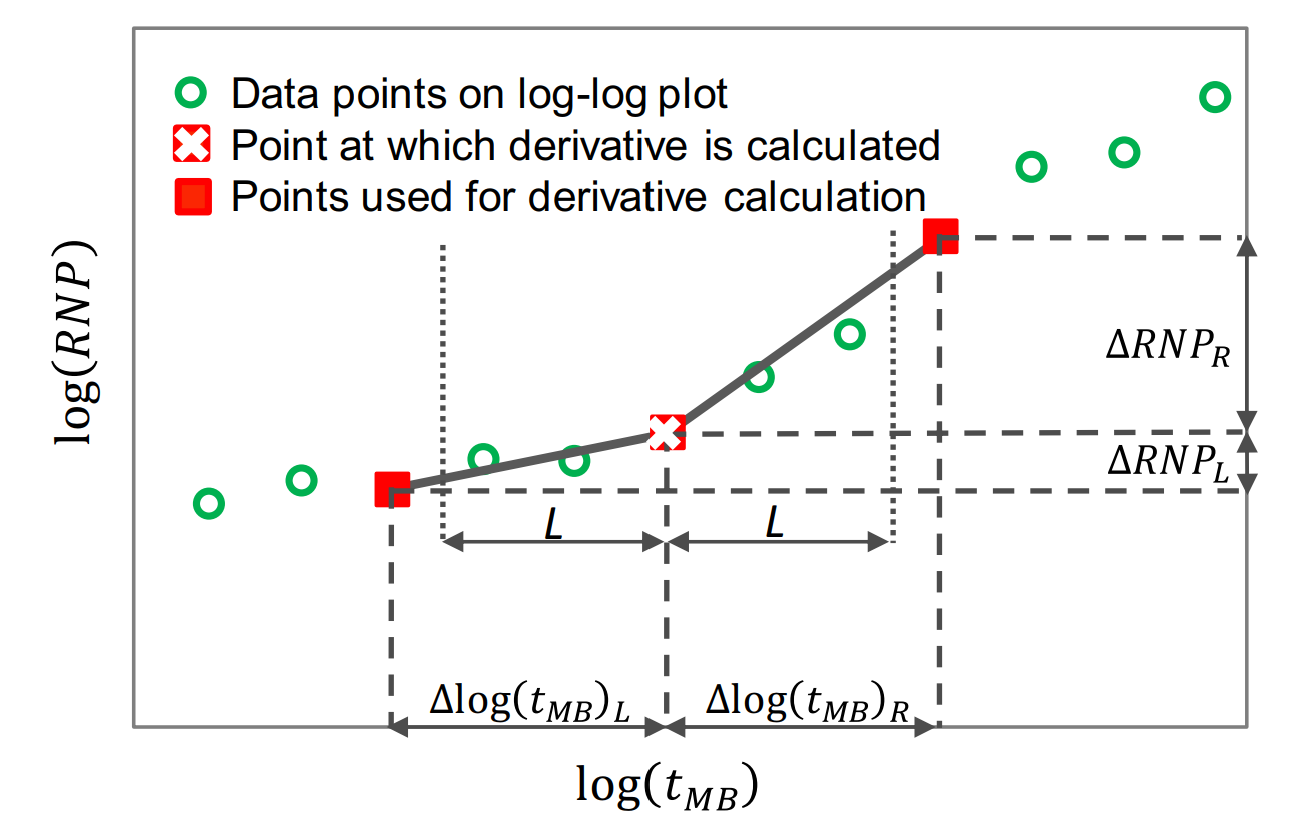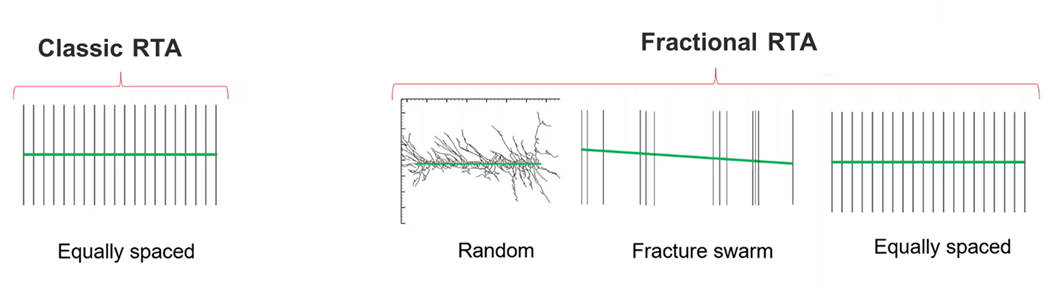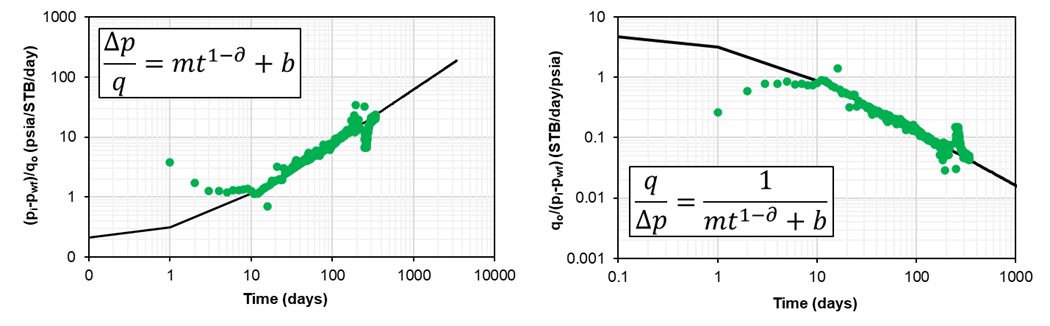# Fractional RTA

## 1. Theory

There have been many studies showing that infinite acting, linear flow is the dominant flow regime in multifractured horizontal wells for tight unconventional reservoirs. Most common production analysis of linear flow was first introduced by Wattenbarger et al. in 1998. They proposed analytical solutions for both constant rate and constant pressure. In reality, rates and pressures are changing simultaneously. To account for that, the principle of superposition is applied.

In 1993, Palacio and Blasingame (1993) introduced the concept of material balance time (MBT). Material balance time

• is a superposition time function.
• converts variable rate data into an equivalent constant rate solution.
• is rigorous for a boundary dominated flow regime
• works well for transient data also, but is only an approximation (errors can be up to 20% for linear flow)

In the whitson+ classical RTA module, material balance time is the default time function, hence the theory for the constant rate solution is emphasized in this part of the manual. On the other hand, there is an option to use constant pressure solution by selecting "Real Time" as a function of time as presented here.

The purpose of conducting this analysis is to determine the linear flow parameters (LFP=$$A\sqrt{k}$$) and drainage volume by interpreting production data. By using Arps equation with respect to time to end of linear flow $$(t_{elf})$$, production forecast can be made.

### 1.1 AssumptionsFig. 1: Wellbox model

Fig.1 shows the geometry of the well used as a base assumption of these analyses. The fracture is assumed to have infinite fracture conductivity, such that the linear flow is perpendicular to the fracture. Additionally, this module assumes single-phase flow with no flow beyond the fracture tip ($$x_e=x_f$$ and $$h=h_f$$), i.e. a fully-penetrating fracture (1D-model).

### 1.2 Superposition Time

There are two superposition time function options in whitson+; material balance time and linear superposition time. In addition to these, one can use real time (called "Variable Pressure" in other tools), in which no superposition time function has been applied.

#### 1.2.1 Material Balance Time - DEFAULT

The use of material balance time is common in the rate transient analysis, and is the default in whitson+. Material balance time

• is a superposition time function.
• converts variable rate data into an equivalent constant rate solution.
• is rigorous for a boundary dominated flow regime
• works well for transient data also, but is only an approximation (errors can be up to 20% for linear flow)

Mathematically, material balance time, MBT $$(t_c)$$ is expressed in:

where $$Q(t)$$ and $$q(t)$$ are cumulative production and production rate at time $$t$$.

#### 1.2.2 Linear Superposition Time

Mathematically, linear superposition time, $$(t_{\text{linear}})$$ is expressed as:

### 1.3 Phase

You can select to run the classical RTA analysis with either of the following rates

• Oil (Surface)
• Gas (Surface)
• Hydrocarbon (Reservoir)
• Liquid (Reservoir)
• Total (Reservoir)

Hydrocarbon reservoir rates are calculated from surface rates using PVT and bottomhole pressures as follows

Liquid reservoir rates are calculated from surface rates using PVT and bottomhole pressures as follows

Total reservoir rates are calculated from surface rates using PVT and bottomhole pressures as follows

In which $$q_{g,res}$$, $$q_{g,res}$$ and $$q_{w,res}$$ can be calculated as follows

The PVT properties solution GOR, $$R_{s}$$, oil formation volume factor, $$B_{o}$$, solution CGR, $$r_{s}$$, gas formation volume factor, $$B_{gd}$$, and water formation volume factor, $$B_{w}$$ is all evaluated at the flowing bottomhole pressure, $$p_{wf}$$, every given day to calculate total rates.

### 1.4 Rate Normalized Pressure (RNP)

Rate Normalized Pressure (RNP) is very useful for production analysis where flowing pressures and rates change through time. It is defined as the flowing pressure drop divided by rate.

### 1.5 Pressure Normalized Rate (PNR)

Pressure normalized rate is the inverse of rate normalized pressure. Pressure normalized rate is very useful for production analysis where flowing pressures and rates change through time. It is defined as the rate divided by flowing pressure drop.

### 1.6 RTA Derivatives

Derivatives assists with flow regime identification and can be calculated for both pressure (p), rate normalized pressure (RNP) and pressure normalized rate (PNR).

Derivative analysis amplifies the reservoir signal, but also amplfies the noise. - Dave Anderson

#### 1.6.1 Derivative Options

The logarithmic derivative used in RTA applied directly on the rate normalized pressure (RNP) data is given by, i.e., .

There are three different ways to compute this derivative in whitson+:

• The Bourdet Derivative (Bourdet et al., 1989)
• Weighted Central Difference
• Central Difference

The Bourdet derivative is commonly used in well-test analysis (PTA) for identifying flow regimes (Lee et al., 2003) and has similarly found utility in RTA.

Governing Equation

To calculate the Bourdet derivative (Bourdet et al., 1989) at any given point, one point before (left) and one point after (right) is used.

Smoothing

The Bourdet approach is illustrated in Fig. 2 and is a way to calculate and smooth the derivative based on a log-cycle window size, L.Fig. 2: Illustration of a derivative calculating using the Bourdet method. Image courtesy of Samaneh Moghadam (April, 2020). Modified from Lee et al. (2003).

It is different from a weighted central difference in the way that it uses a constant log-cycle window size, L, on both sides of the point of derivation. A weighted central difference, on the other hand, uses a constant index step size, e.g. i-5 and i+5 for step size of 5, on each side of the point of derivation. The window size, L, represents the log-cycle fraction used to control the amount of smoothing. Typical values are 0.01 to 0.2. The default L in whitson+ is 0.2. The larger the L value, the more smoothing.

The Bourdet algorithm implemented in whitson+ was provided by Behnam Zanganeh.

#### 1.6.3 Weighted Central Difference

The weighted central difference is given by,

where the $$L$$-subscript refers to the index to the left with a constant step size, $$S$$, i.e. $$i-S$$ and the $$R$$-subscript refers to the index to the right with a constant step size, $$S$$, i.e. $$i+S$$.

#### 1.6.4 Central Difference

This is the naive implementation, given by three points per derivative for central difference in the following manner

#### 1.6.5 Derivatives in Material Balance Time

Because of noise in real field data, material balance time may not be in sequence. This might cause problems in derivative calculations. Hence, the data must be sorted in terms of increasing material balance time prior to calculating the derivative. The following procedure, adapted from Samandarli et al.(2012), is used for this purpose.

1. RNP and material balance time $$(t_c)$$ are calculated at each real time step.
2. RNP and $$(t_c)$$ are sorted in increasing order of material balance time, with repeat material balance times eliminated.
3. The RNP derivative, RNP', is then calculated using the sorted data.

### 1.7 RTA Integrals

In the case of complex and noisy data, Blasingame et al.  introduced the concepts of pressure-integral and pressure-integral difference which have become essential tools in scrutiny of well-test data throught type-curve analysis. Similarly, McCray (1990) presented the idea of the rate-integral and rate-integral derivative which was utilized by Blasingame and his students and colleagues for type-curve analysis of production data in various publications.

### 1.7.1 The Rate Normalized Pressure (RNP) Integral

The use of integral of the RNP $$\left(IRNP\right)$$ and its derivative $$\left(IRNP'_R\right)$$ was suggested by Chu et al.  for the study of flow-regime signature in the Wolfcamp shale. For liquids, the equations are written as follows:

The integral can be calculated using various time functions (real time, linear superposition time). However, it is presented here in material balance time for example purposes.

As with $$\left(RNP\right)$$ and $$\left(RNP'_R\right)$$ the calculations for $$\left(IRNP\right)$$ and $$\left(IRNP'_R\right)$$ can be applied to gases through inclusion of gas pseudovariables.

### 1.7.2 Discrete Calculation

The discrete form of Eq. \eqref{eq:RTA-integral} is expressed in the following manner:

The conversion from a slope to LFP (A√k) while using the RNP integral must be adjusted by 2/3 to ensure that the LFP is calculated correctly from the slope. This follows from integrating the RNP as a function of √t.

When turning on the integral function, this correction is applied

### 1.8 Flow Regimes

To understand why it is important to account for uneven fracture spacing, we repeat the three relevant flow regimes tight unconventionals.

1. Infinite acting flow, often referred to as transient flow, is the flow regime that ends as the pressure transient reaches one reservoir boundary.

2. Transitional flow is the flow regime starts as the pressure transient reaches one reservoir boundary and ends when the pressure propagation reaches all reservoir boundaries.

3. Boundary dominated flow (∂p/∂t=constant at all locations), also called pseudo-stedy state, is the flow regime that starts as the pressure propagation reaches all reservoir boundaries. It occurs when all outer boundaries of the reservoir are no-flow boundaries. These boundaries can be both sealing faults and nearby producing wells or fractures. During this period, the change in pressure at any place in the reservoir decreases at the same, constant rate. The reservoir is said to behave as a “tank”.

For a well geometry with uneven fracture spacing, the flow regime is 1) infinite acting until the boundary between the fractures with the smallest spacing is observed, 2) thereafter it is transitional flow until the boundary between the fractures with the largest spacing is observed, and 3) after that it is in full boundary dominated flow.

### 1.9 Difference between Classical and Fractional RTAFig. 3: Visual of the difference between classic RTA and fractional RTA. Courtesy: Jorge Acuna.

In whitson+, we distinguish between classical RTA and fractional RTA. Classical RTA solves for equally spaced fracture networks and resolves LFP = A√k = $$Ak^{0.5}$$ (δ = 0.5). Fractional RTA solves for complex system of fractures and resolves LFP = $$Ak^δ$$ (any value of δ). A visual representation of the difference is shown in Fig. 3. Acuna’s (2016, 2020) δ-parameter is a measure how “uneven” the fracture spacing is. For infinite conductive fracture, the δ value is between 0 (highly uneven fractures) and 0.5 (even fracture spacing).

### 1.10 Estimate δ from Production DataFig. 4: Visual of the difference between classic RTA and fractional RTA. Courtesy: Jorge Acuna.

An estimate of δ can be obtained by plotting rate normalized pressure (RNP) versus time, [(pi-pwf)/q vs. t on a log-log scale] followed by a best fit of the power-law function provided by

Alternatively, one can plot and fit the reciprocal, i.e., the pressure normalized rate (PNR) versus time [q/(pi-pwf) vs. t]. Examples of these plots are provided in Fig. 4. For single-phase oil cases (e.g., $$p_{wf}$$ > $$p_{b}$$, little/no water) oil rates can be used. For multiphase systems ($$p_{wf}$$ < $$p_{sat}$$ and/or significant water production), it is recommended to use total reservoir rates (surface oil, gas and water rates converted from surface to reservoir volumes using proper PVT and pressures). For single-phase gas (dry/wet gas or $$p_{wf}$$ > $$p_{dew}$$, little/no water) one can use gas rates and pseudopressure.

### 1.11 Physical significance of δ

In material balance time,

• δ = 0.75 indicates bi-linear flow
• δ > 0.5 indicates low conductivity fracture systems
• δ = 0.5 indicates infinite acting linear flow
• 0 < δ < 0.5 indicates transitional flow
• δ = 0 indicates boundary dominated flow

Why do we generally prefer to use material balance time for analyzing δ-parameter in Fractional RTA?

• Infinite acting linear flow, in real time and material balance time, exhibits a δ of 0.5.
• In material balance time, transitional flow will exhibit a δ between 0 and 0.5 and boundary dominated flow yields δ = 0 (a unit slope on the RNP vs MBT plot).
• In real time, however, transitional flow δ ranges from 0.5 to -∞ and boundary dominated flow yields
δ = -∞ . Hence, using this range to analyze flow regimes may be impractical for most people.

### 1.12 Relationship between δ and Arps' b-factor

There is a relationship between the δ-parameter and Arps' b-factor. Remember that if you use the δ-parameter parameter to constrain b-factors used in DCA, you must resolve the δ-parameter in real time (not material balance time).

### 1.13 Governing Equations

The equation for rate normalized pressure is written as

where $$m$$ is the slope of RNP versus time.

For oil:

For gas:

Constant flowing pressure solution:

Constant rate solution:

For gas, use pseudopressure to substitue $$p_i$$ and $$p_{wf}$$ in Eqs. \eqref{eq:cp-solution} and \eqref{eq:cr-solution}.

The equation for skin (S) is related to the intercept b, and given by

Here $$x_f$$ is the cumulative length of the fracture.

### 1.14 Solving for LFP

One can solve for LFP by knowing the estimates of m (coefficient) and δ (slope) obtained from the rate normalized pressure (RNP) versus time plot, [(pi-pwf)/q vs. t on a log-log scale].

For oil:

For gas: